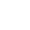﻿

/    2018-09-03

Go语言切片是对数组的抽象。数组的长度不可改变，在特定场景中这样的集合就不太适用，Go中提供了一种灵活，功能强悍的内置类型切片("动态数组")，与数组相比切片的长度是不固定的，可以追加元素，在追加时可能使切片的容量增大。

`var slice []int`

```var slice0 []int = []int{1, 2, 3}
var slice1 = []int{1, 2, 3}```

```var slice0 []int = make([]int, 10)
var slice1 = make([]int, 10)
var slice2 = make([]int, 10, 10)```

```slice0 := []int{}
slice1 := make([]int, 10)
slice2 := make([]int, 10, 10)```

```var array = [...]int{0, 1, 2, 3, 4, 5, 6, 7, 8, 9}
var slice0 []int = array[2:8]
// 可以简写为 var slice []int = array[:end]
var slice1 []int = array[0:6]
// 可以简写为 var slice[]int = array[start:]
var slice2 []int = array[5:10]
// 可以简写为var slice []int = array[:]
var slice3 []int = array[0:len(array)]
// 去掉切片的最后一个元素
var slice4 = array[:len(array)-1]```

```package main
import (
"fmt"
)
func main() {
slice := []int{0, 1, 2, 3, 4, 5, 6, 7, 8, 9}
slice1 := slice[6:8]
fmt.Printf("slice1 : %v , len : %d , cap : %d\n", slice1, len(slice1),
cap(slice1))
slice2 := slice[2:6:8]
fmt.Printf("slice2 : %v , len : %d , cap : %d\n", slice2, len(slice2),
cap(slice2))
}```

slice1[6:8]，从第6位到第8位(返回[6 7])，长度len为2，最大可扩充长度cap为4

slice2[2:6:8]，从第2位到第6位(返回[2 3 4 5])，长度len为4，最大可扩充长度cap为6

```package main
import "fmt"
func main() {
// 通过初始化表达式构造，可使用索引号。
s1 := []int{0, 1, 2, 3, 8: 100}
fmt.Println(s1, len(s1), cap(s1))
// 使用 make 创建，指定 len 和 cap 值。
s2 := make([]int, 6, 8)
fmt.Println(s2, len(s2), cap(s2))
// 省略 cap，相当于 cap = len。
s3 := make([]int, 6)
fmt.Println(s3, len(s3), cap(s3))
}```

```package main
import (
"fmt"
)
func main() {
var a = []int{1, 2, 3}
// 一次 append 一个值
b := append(a, 4)
// 一次 append 多个值
c := append(b, 5, 6, 7)
// 一次 append 一个切片
var d = []int{8, 9, 10}
e := append(c, d...)
fmt.Println(a, b, c, d, e)
}```

```package main
import (
"fmt"
)
func main() {
var a = []int{1, 2, 3, 4, 5}
b := []int{100, 200}
copy(a, b)
fmt.Println(a, b)
}```

```[100 200 3 4 5] [100 200]
package main
import (
"fmt"
)
func main() {
var a = []int{1, 2, 3, 4, 5}
b := []int{100, 200}
copy(b, a)
fmt.Println(a, b)
}```

`[1 2 3 4 5] [1 2]`

slice中cap重新分配规律：

```package main
import (
"fmt"
)
func main() {
s := make([]int, 0, 1)
c := cap(s)
for i := 0; i < 50; i++ {
s = append(s, i)
if n := cap(s); n > c {
fmt.Printf("cap: %d -> %d\n", c, n)
c = n
}
}
}```

```cap: 1 -> 2
cap: 2 -> 4
cap: 4 -> 8
cap: 8 -> 16
cap: 16 -> 32
cap: 32 -> 64```(2)

﻿